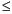Functions and CALL Routines

# BETAINV Function

Returns a quantile from the beta distribution.
 Category: Quantile

## Syntax

 BETAINV (p,a,b)

### Arguments

p

is a numeric probability.

 Range: 0p1
a

is a numeric shape parameter.

 Range: a > 0
b

is a numeric shape parameter.

 Range: b > 0

The BETAINV function returns the pth quantile from the beta distribution with shape parameters a and b. The probability that an observation from a beta distribution is less than or equal to the returned quantile is p.

Note:   BETAINV is the inverse of the PROBBETA function.SAS Statements Results
`x=betainv(0.001,2,4);`
`0.0101017879`

 Functions:Previous Page | Next Page | Top of Page